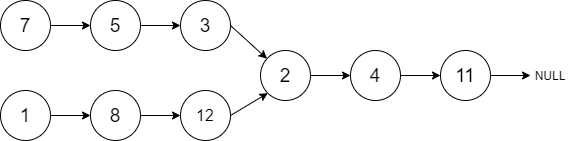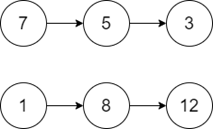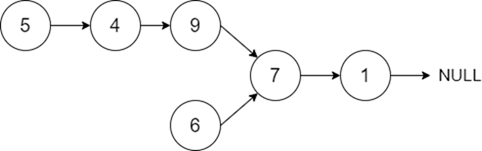# Find the Intersection Point of Two Linked Lists in Java

A Linked List is a linear data structure in which each node has two blocks such that one block contains the value or data of the node and the other block contains the address of the next field.

Let us assume that we have a linked list such that each node contains a random pointer which is pointing to the other nodes in the list. The task is to find the node at which two linked lists intersect each other. If they don’t intersect, then return NULL or empty as output.

For Example

Input-1:Output:

2

Explanation: Since the given linked list intersects at the node with the value ‘2’, we will return the value ‘2’ as the output.

Input-2:Output:

NULL

Explanation: Since there are no common points, we will return NULL in this case.

## Approach to Solve this Problem

We have two linked lists with a common point where they intersect each other. To find the intersection point, we will traverse both the linked lists till we find that they are equally pointing to the same value. At some point, the pointer to the next node of the linked list will be the same. Thus we will return the value of that point.

• Take two linked lists with data and pointer to the next node.
• A function commonPoint(listnode*headA, listnode*headB) takes two pointers of linked list respectively and returns the value of the common or intersection point of the linked list.
• An integer function that finds the length of the linked list will return the length of both linked lists from the head of the list.
• Now create a pointer to the head of both lists and traverse the list which is greater in its length till (length of first list – length of second list).
• Now traverse the list till we find the next pointer is equal.
• Return the value of that particular node where both the lists intersect.

## Example

Live Demo

public class Solution {
static class listnode {
int data;
listnode next;
listnode(int d) {
data = d;
next = null;
}
}
int c = 0;
c++;
}
return c;
}
if (c1 > c2) {
for (int i = 0; i < c1 - c2; i++) {
if (p1 == null) {
return - 1;
}
p1 = p1.next;
}
}
if (c1 < c2) {
for (int i = 0; i < c2 - c1; i++) {
if (p2 == null) {
return - 1;
}
p2 = p2.next;
}
}
while (p1 != null &amp;&amp; p2 != null) {
if (p1.data == p2.data) {
return p1.data;
}
p1 = p1.next;
p2 = p2.next;
}
return - 1;
}
public static void main(String[] args) {
Solution list = new Solution();
}
}

Running the above code will generate the output as,

## Output

7

Explanation: The given linked lists are intersecting at 7.Updated on: 23-Feb-2021

427 Views